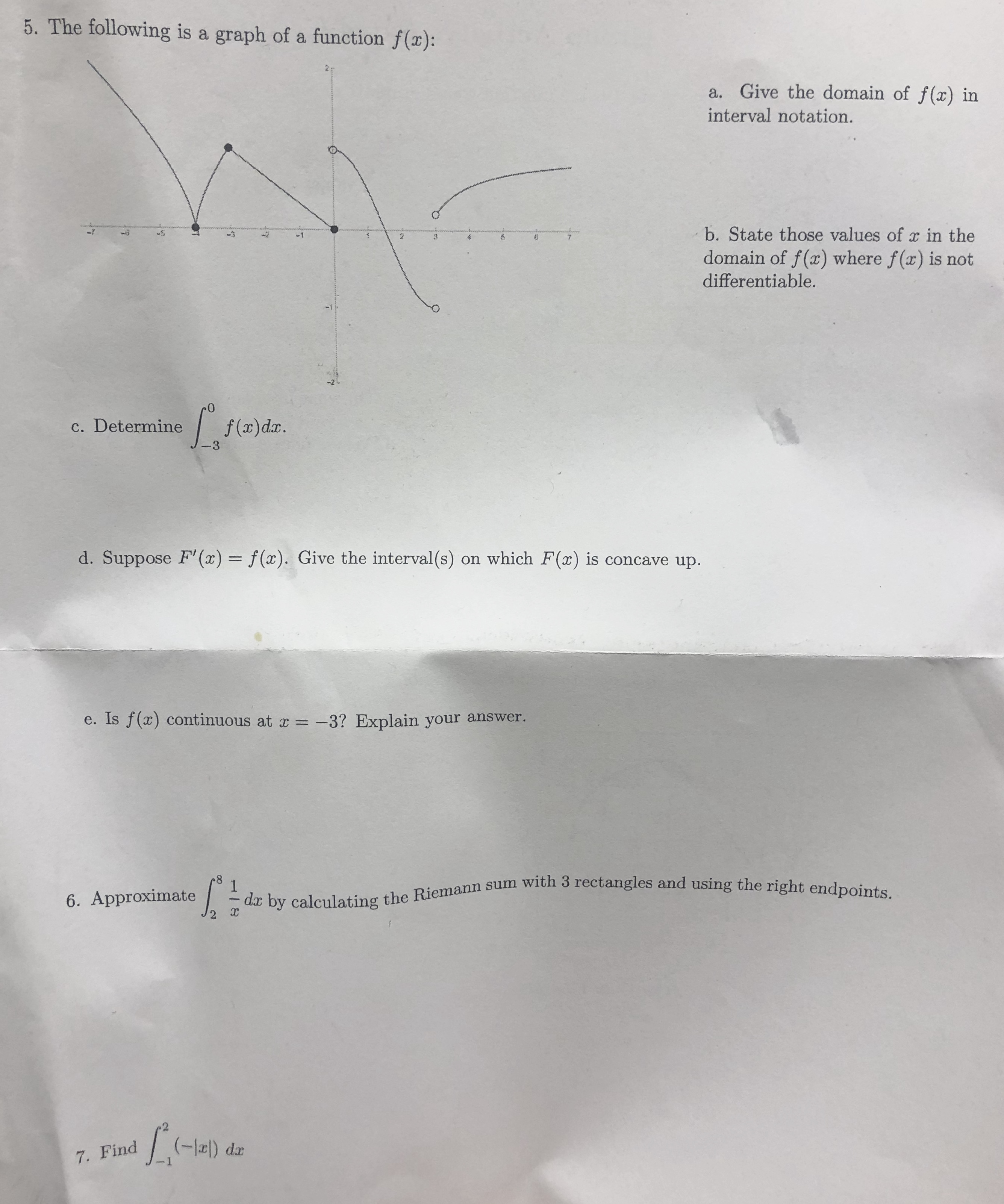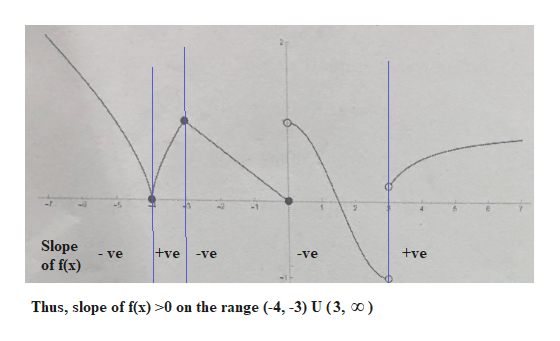# 5. The following is a graph of a function f(x):a. Give the domain of f(x) ininterval notation.b. State those values of r in the-337domain of f(x) where f(x) is notdifferentiable.efa)da.c. Determine-3d. Suppose F' (x) = f(x). Give the interval(s) on which F(x) is concave up.e. Is f(x) continuous at x-3? Explain your answer.- da by calculating the Riemann sum with 3 rectangles and using the right endpoints.16. Approximate7. Find

Question
1 views

Parts d and ehelp_outlineImage Transcriptionclose5. The following is a graph of a function f(x): a. Give the domain of f(x) in interval notation. b. State those values of r in the -3 3 7 domain of f(x) where f(x) is not differentiable. efa)da. c. Determine -3 d. Suppose F' (x) = f(x). Give the interval(s) on which F(x) is concave up. e. Is f(x) continuous at x -3? Explain your answer. - da by calculating the Riemann sum with 3 rectangles and using the right endpoints. 1 6. Approximate 7. Find fullscreen
check_circle

Step 1

F(x) will be concave upwards when F''(x) > 0.

Now, F''(x) can be found from the graph of f(x)

Since, we are only interesed in sign changes, we can easily find it on the graph of f(x), as shown below:help_outlineImage TranscriptioncloseSlope of f(x) +ve +ve -ve - ve ve Thus, slope of f(x) >0 on the range (-4, -3) U (3, ao) fullscreen
Step 2

(d) Thus, the interval on which F(x) is concave up ...

### Want to see the full answer?

See Solution

#### Want to see this answer and more?

Solutions are written by subject experts who are available 24/7. Questions are typically answered within 1 hour.*

See Solution
*Response times may vary by subject and question.
Tagged in

### Calculus